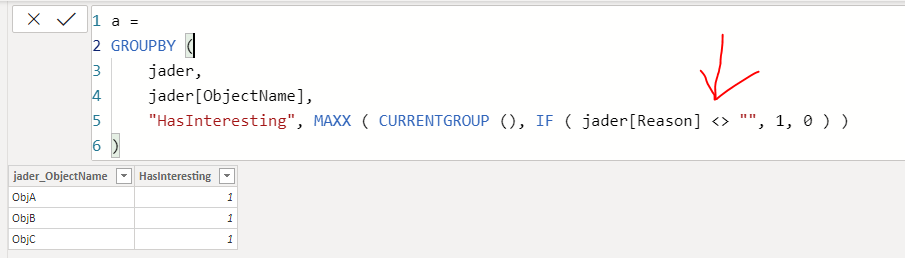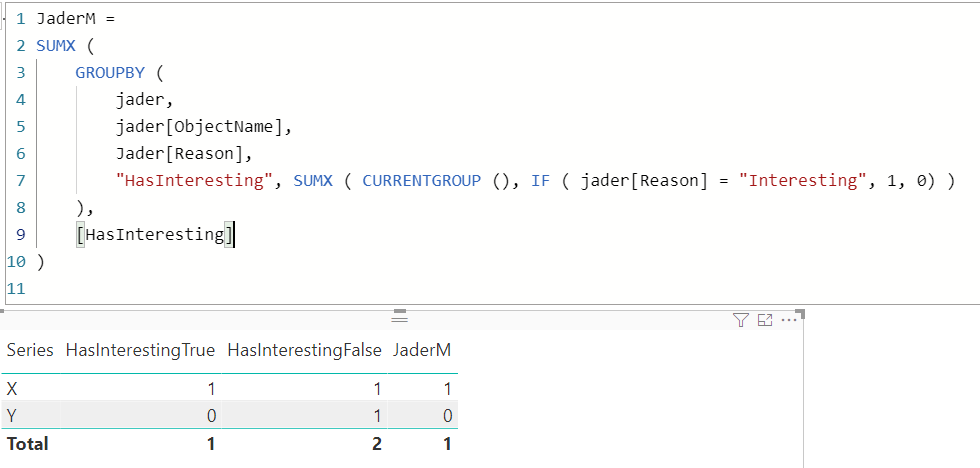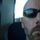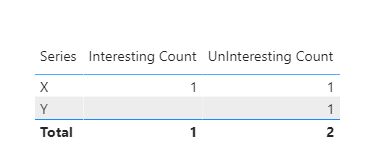cancel
Showing results for
Did you mean:

Fabric is Generally Available. Browse Fabric Presentations. Work towards your Fabric certification with the Cloud Skills Challenge.Microsoft

## A Measure that depends upon the result of another measure

I have rows where certain values are interesting, and I want to split the data into HasInteresting with counts for true or false. The trick is that it's not if the row is interesting, but if any row in a certain grouping has an interesting row.

So if I have something like the below:

 ObjectName Series Reason Duration ObjA X Mild 12 ObjA X Interesting 454 ObjB Y Mediocre 12 ObjB Y Mild 32 ObjC X Mild 87 ObjC X Boring 37

I've added a calculated column of IsInteresting = IF([Reason] = "Interesting", 1, 0), and then a measure of HasInteresting = SUM([IsInteresting]) > 0. Which generates the following correct result.

 ObjectName HasInteresting ObjA True ObjB False ObjC False

But what I want to know is "How many ObjectName's have an HasInteresting of True, and how many have False by Series".

It would look something like

 Series HasInterestingTrue HasInterestingFalse X 1 1 Y 0 1

I tried doing something with COUNTROWS, but that returned results from the original rows, not from the middle table.

1 ACCEPTED SOLUTIONCommunity Champion

``````HasInterestingTrue =
CALCULATE (
COUNTROWS ( DISTINCT ( Jader[ObjectName] ) ),
) + 0
``````
``````HasInterestingFalse =
VAR ObjectThatHasInteresting =
VAR Result =
CALCULATE (
COUNTROWS ( DISTINCT ( Jader[ObjectName] ) ),
)
RETURN
Result
``````17 REPLIES 17Community Champion

``````HasInterestingTrue =
CALCULATE (
COUNTROWS ( DISTINCT ( Jader[ObjectName] ) ),
) + 0
``````
``````HasInterestingFalse =
VAR ObjectThatHasInteresting =
VAR Result =
CALCULATE (
COUNTROWS ( DISTINCT ( Jader[ObjectName] ) ),
)
RETURN
Result
``````Microsoft

This works. But now that I have your attention, I would like your thoughts on where my thinking has gone wrong with something I was trying a few hours ago. I thought that I could create a Measure using GROUPBY and make use of that.

``Measure = GROUPBY(jader, jader[ObjectName], "HasInteresting", MAXX(CURRENTGROUP(), IF(jader[Reason], 1, 0)))``

The above errors out with "The expression refers to multiple columns. Multiple columns cannot be converted to a scalar value". What am I not understanding about GROUPBY?Community Champion

@jader3rd  GROUPBY is a table function, your measure returns the below table, and implicitly DAX can only convert 1 row and 1 column table into a scalar value. Hence your measure throws an error.

Also had to do a comparision inside IF otherwise it won't work.Microsoft

Okay. I normally figure out the formulas by solving an inner part and then wrapping it in the next level. That approach apparently doesn't work with GROUP BY.

What would I wrap the GROUP BY in to make the Measure?Community Champion

@jader3rd That's where things always go wrong, I have stopped thinking of DAX as excel formulas or any other programming language, because you have to think holistically and not just from inside out.

GROUPBY is useful when you have created temporary columns on the fly and you want to summarize the result by those columns but aren't able to do so because they do not carry the data lineage of a column of the model. CURRENTGROUP helps in accessing the current row context and then you can apply SUMX, COUNTX or any either iterator to get the result for the values created virtually.

But here is how you can make your version work.

``````JaderM =
SUMX (
GROUPBY (
"HasInteresting", SUMX ( CURRENTGROUP (), IF ( jader[Reason] = "Interesting", 1, 0 ) )
),
[HasInteresting]
)
``````Microsoft

That does get me the count for HasInteresting, but it's not working for the count of objects names with no interesting reasons.

I tried

``Measure2 = COUNTROWS( FILTER(GROUPBY (jader, jader[ObjectName], jader[Reason], "HasInteresting", MAXX( CURRENTGROUP(), IF (jader[Reason] = "Interesting", 1, 0))), [HasInteresting] = 0))``

But that gives the count of the underlying rows that doesn't have interesting. Which boggles my mind. The GROUPBY should group on object name and return a 3 row table to the Filter. The Filter statement should then filter out the single row with HasInteresting = 1, and return two rows. The COUNTROWS should then count the two remaining rows. Obviously I have something backwards.Community Champion

You can try to debug your measure step by step in a table and you can see the rows returned by GROUPBY to FILTERMicrosoft

I see what I did wrong now. I put jader[Reason] in the GROUP BY, even though I shouldn't have. Now it all makes sense.Super User

Different way of thinking @AntrikshSharma 👍

My approach was to calculate each one the same way and just look at the results of the calculated table. But the hard part was getting any object name that had an "interesting" in it and excluding it for all same object names that didn't. You just set that to a variable, then excluded it from your final results.

Did my answers help arrive at a solution? Give it a kudos by clicking the Thumbs Up!

DAX is for Analysis. Power Query is for Data Modeling

Proud to be a Super User!

MCSA: BI ReportingMicrosoft

Thanks for your trial and error with this @edhans. It means a lot to me.Super User

Thanks for your trial and error with this @edhans. It means a lot to me.

The approach of @AntrikshSharma is really common sense from the standpoint of build result a, then result b and exclude result a from b.

But I was so far down the path mentally of building the tables in my original solution, I didn't see the forest for the trees. 😁

But I did get a blog post out of it. Found out some weird behavior using NOT as an operator and function, which it can do both, so all is not lost.

Kudos appreciated, and glad you have a working solution!

Did my answers help arrive at a solution? Give it a kudos by clicking the Thumbs Up!

DAX is for Analysis. Power Query is for Data Modeling

Proud to be a Super User!

MCSA: BI ReportingSuper User

@jader3rd I would try to avoid the intermedary tables and calculated columns if possible. Try something like this, which is a single measure:

``````Interesting Count =
VAR varInterstingCount =
COUNTX(
FILTER(
'Table',
'Table'[Reason] = "Interesting"
),
'Table'[Reason]
)
RETURN
COALESCE(varInterstingCount, 0)``````In general, try to avoid calculated columns. There are times to use them, but it is rare. Getting data out of the source system, creating columns in Power Query, or DAX Measures are usually preferred to calculated columns. See these references:
Calculated Columns vs Measures in DAX
Calculated Columns and Measures in DAX
Storage differences between calculated columns and calculated tables
SQLBI Video on Measures vs Calculated Columns

Does that help?

Did my answers help arrive at a solution? Give it a kudos by clicking the Thumbs Up!

DAX is for Analysis. Power Query is for Data Modeling

Proud to be a Super User!

MCSA: BI ReportingMicrosoft

That gets me the interesting count, but I also need the uninteresting. I am trying to do a comparison of how many Objects have any rows which are interesting, vs how many do not have any interesting rows.Super User

Just a tweak and 2nd measure @jader3rd

``````UnInteresting Count =
VAR varInterstingCount =
COUNTX(
FILTER(
'Table',
'Table'[Reason] <> "Interesting"
),
'Table'[Reason]
)
RETURN
COALESCE(varInterstingCount, 0)``````Did my answers help arrive at a solution? Give it a kudos by clicking the Thumbs Up!

DAX is for Analysis. Power Query is for Data Modeling

Proud to be a Super User!

MCSA: BI ReportingMicrosoft

That's still the Count of Rows, not the Count of ObjectNames which do contain an interesting Reason, and those which don't at all. The total sum in the final table should be 3, because there are 3 ObjectNames.Super User

Ok @jader3rd - I didn't understand fully the original request. So this is a bit longer,but still two measures.

``````Interesting Count =
COUNTX(
FILTER(
SUMMARIZE(
'Table',
'Table'[ObjectName],
'Table'[Series]
),
"Reasons",
CONTAINSSTRING(
CALCULATE(
CONCATENATEX(
'Table',
'Table'[Reason],
", "
)
),
"Interesting"
) * 1
),
[Reasons] = 1
),
[Reasons]
)``````

and

``````UnInteresting Count =
COUNTX(
FILTER(
SUMMARIZE(
'Table',
'Table'[ObjectName],
'Table'[Series]
),
"Reasons",
CONTAINSSTRING(
CALCULATE(
CONCATENATEX(
'Table',
'Table'[Reason],
", "
)
),
"Interesting"
) * 1
),
[Reasons] = 0
),
[Reasons]
)``````It does this by building a virtual table first that looks like this:which comes from this - you can see this in DAX Studio, or you could create a new calculated table with this code to see it:

``````ADDCOLUMNS(
SUMMARIZE(
'Table',
'Table'[ObjectName],
'Table'[Series]
),
"Reasons",
CONTAINSSTRING(
CALCULATE(
CONCATENATEX(
'Table',
'Table'[Reason],
", "
)
),
"Interesting"
)
)``````then the rest just converts the true/false to 1/0, then it counts those.

I do wonder if @Greg_Deckler or @TomMartens could shorten this up a bit though.

Did my answers help arrive at a solution? Give it a kudos by clicking the Thumbs Up!

DAX is for Analysis. Power Query is for Data Modeling

Proud to be a Super User!

MCSA: BI ReportingSuper User

Wow... my spelling is horrible.... 😂

Did my answers help arrive at a solution? Give it a kudos by clicking the Thumbs Up!

DAX is for Analysis. Power Query is for Data Modeling

Proud to be a Super User!

MCSA: BI ReportingAnnouncements#### Power BI Monthly Update - November 2023

Check out the November 2023 Power BI update to learn about new features.#### Fabric Community News unified experience

Read the latest Fabric Community announcements, including updates on Power BI, Synapse, Data Factory and Data Activator.#### The largest Power BI and Fabric virtual conference

130+ sessions, 130+ speakers, Product managers, MVPs, and experts. All about Power BI and Fabric. Attend online or watch the recordings.Top Solution Authors
Top Kudoed Authors
Users online (4,711)